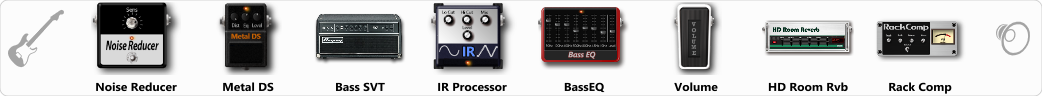# Black Metal Aggro Bass

Discussion in 'ToneLib-GFX presets' started by Tetragramm1983, Jan 15, 2021.

1. ### Tetragramm1983Active Member

Black Metal Aggro Bass

Preset name: Black Metal Bass

Effects chain:Effect: "Noise Reducer" (Dynamics / Filter), active - "yes"
"Sens" = 88
"Mode" = Hard

Effect: "Metal DS" (Overdrive / Distortion), active - "yes"
"Dist" = 35
"Bass" = 85
"Middle" = 60
"Treble" = 45
"Level" = 55

Effect: "Bass SVT" (Amp simulators), active - "yes"
"Bass Ctrl" = UltraLo
"Midrange" = 800 Hz
"Ultra Hi" = On
"Bass" = 2
"Middle" = 0
"Treble" = -2
"Volume" = 70
"Level (dB)" = -6

Effect: "IR Processor" (Dynamics / Filter), active - "yes"
"IR" = Aggressive Cab Cn.A1
"Low Cut (Hz)" = 118
"Hi Cut (kHz)" = 12.5
"Mix" = 85
"Level (dB)" = 2

Effect: "BassEQ" (Dynamics / Filter), active - "yes"
"50 Hz" = -8
"120 Hz" = -8
"400 Hz" = -12
"500 Hz" = 4
"800 Hz" = -6
"4.5 kHz" = 4
"10 kHz" = 4
"Level (dB)" = -2

Effect: "Volume" (Dynamics / Filter), active - "yes"
"Pedal Pos." = 100
"Pan" = 0

Effect: "HD Room Rvb" (Reverb), active - "yes"
"Time" = 7.3
"PreLPF" = 100
"PreDelay" = 88
"HiDamp" = 55
"LoGain" = -6.0
"Mix" = 45

Effect: "Rack Comp" (Dynamics / Filter), active - "yes"
"Threshold (dB)" = -24
"Ratio" = 3
"Attack" = Fast
"Release (ms)" = 300
"Knee" = 9
"Level (dB)" = 10

Note: You will need to download and install the ToneLib-GFX software to use the preset.

#### Attached Files:

• ###### Black_Metal_Aggro_Bass.tlgfx
File size:
4.2 KB
Views:
2,917
oNeKOG, The MASK, pavelkoniak and 5 others like this.